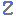Visit Zacobria Webshop

Client-Interfaces – reading the cartesian coordinates (sometimes reffered to as matlab data).

Application Description:
This example shows the use of client-Interfaces (Also sometimes reffered to as Matlab data) on the Universal-Robots to read the robot position from external device.

The application uses the port 30003 on the Universal-Robots which is streaming data out containing informations about the robot status. These data might look like random unreadable data similar to data in a binary file, but thats because the way the data are formatted.

Below is a screen shoot of the dataflow streamed out from port 30003.

Port 30003 is part of the Client-Interfaces and an overview can be found at this link. Client-Interfaces document

In the file Client-Interface.xls at the tab RealTime_3.2 and overview of the data streamed out can be found and where each data can be found in the data stream.

Notice the data for cartesian coordinates shown in line 14 is of type double and has a size of 48 bytes.

Function description:

The aim is to read the Cartiseian coordinates of the tool (X, Y, Z, Rx, Ry, Rz).

(This is similar to reading these data from the MODBUS registers as described in this article MODBUS example of reading the Cartesian position data.)

In this case the cartesian coordinate data are read from a external host with Python code.

Program description:
The external host connects to port 30003 on the UR and continually reads the dataflow which the robot stream out and convert the raw data to readable data in mm.

Since the desired data is located some way down the list as shown in client-interfaces.xls document – therefore the previous data are read first, but not converted and shown.

This means blocks of 4 + 8 + 48 + 48 + 48 + 48 + 48 + 48 + 48 + 48 + 48 are read first, but not converted and not shown.

The next block of 48 bytes contain the data desired – and since the data consists of 6 values (each value therefore contain 8 bytes) then each value of 8 bytes are read one by one and printed out to the screen.

The data are formatted as double and therefore they are converted in human readable data. Some more informations about double can be found at this link Double-precision floating-point format.

Program code:

No program on UR.

Host program in Python code.

```# Echo client program
import socket
import time
import struct

HOST = "192.168.0.9" # The remote host
PORT_30003 = 30003

print "Starting Program"

count = 0
home_status = 0
program_run = 0

while (True):
if program_run == 0:
try:
s = socket.socket(socket.AF_INET, socket.SOCK_STREAM)
s.settimeout(10)
s.connect((HOST, PORT_30003))
time.sleep(1.00)
print ""
packet_1 = s.recv(4)
packet_2 = s.recv(8)
packet_3 = s.recv(48)
packet_4 = s.recv(48)
packet_5 = s.recv(48)
packet_6 = s.recv(48)
packet_7 = s.recv(48)
packet_8 = s.recv(48)
packet_9 = s.recv(48)
packet_10 = s.recv(48)
packet_11 = s.recv(48)

packet_12 = s.recv(8)
packet_12 = packet_12.encode("hex") #convert the data from \x hex notation to plain hex
x = str(packet_12)
x = struct.unpack('!d', packet_12.decode('hex'))
print "X = ", x * 1000

packet_13 = s.recv(8)
packet_13 = packet_13.encode("hex") #convert the data from \x hex notation to plain hex
y = str(packet_13)
y = struct.unpack('!d', packet_13.decode('hex'))
print "Y = ", y * 1000

packet_14 = s.recv(8)
packet_14 = packet_14.encode("hex") #convert the data from \x hex notation to plain hex
z = str(packet_14)
z = struct.unpack('!d', packet_14.decode('hex'))
print "Z = ", z * 1000

packet_15 = s.recv(8)
packet_15 = packet_15.encode("hex") #convert the data from \x hex notation to plain hex
Rx = str(packet_15)
Rx = struct.unpack('!d', packet_15.decode('hex'))
print "Rx = ", Rx

packet_16 = s.recv(8)
packet_16 = packet_16.encode("hex") #convert the data from \x hex notation to plain hex
Ry = str(packet_16)
Ry = struct.unpack('!d', packet_16.decode('hex'))
print "Ry = ", Ry

packet_17 = s.recv(8)
packet_17 = packet_17.encode("hex") #convert the data from \x hex notation to plain hex
Rz = str(packet_17)
Rz = struct.unpack('!d', packet_17.decode('hex'))
print "Rz = ", Rz

home_status = 1
program_run = 0
s.close()
except socket.error as socketerror:
print("Error: ", socketerror)
print "Program finish"

```

Program run:

The robot is in this position – Notice the X, Y, Z, Rx, Ry, Rz values in the Move screen.

Below is the printout on the screen with the X, Y, Z, Rx, Ry, Rz values when the Python program is executed. The values are collected from port 30003 and correspond with the actual values of the robot position. The X, Y, Z are in mm and Rx, Ry, Rz are in radians.

And this is also the position of the robot.

Notice: Data of a very small value is written as exponent of 10 – and a value of 10-05 is very small – and is virtual 0.

X = 0.0
Y = 300.0
Z = 300.0
Rx = 0.0
Ry = 3.14
Rz = 0.0

Disclaimer: While the Zacobria Pte. Ltd. believes that information and guidance provided is correct, parties must rely upon their skill and judgement when making use of them. Zacobria Pte. Ltd. assumes no liability for loss or damage caused by error or omission, whether such an error or omission is the result of negligence or any other cause. Where reference is made to legislation it is not to be considered as legal advice. Any and all such liability is disclaimed.

If you need specific advice (for example, medical, legal, financial or risk management), please seek a professional who is licensed or knowledgeable in that area.

Author:
By Zacobria Lars Skovsgaard
Accredited 2015-2018 Universal Robots support Centre and Forum.

Tweet